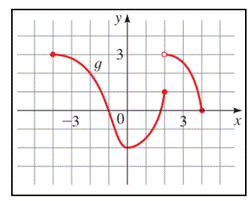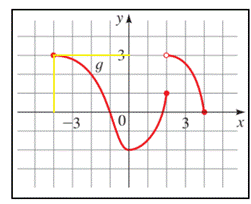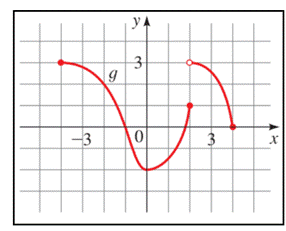# the values of g ( − 4 ) , g ( − 2 ) , g ( 0 ) , g ( 2 ) and g ( 4 )### Precalculus: Mathematics for Calcu...

6th Edition
Stewart + 5 others
Publisher: Cengage Learning
ISBN: 9780840068071### Precalculus: Mathematics for Calcu...

6th Edition
Stewart + 5 others
Publisher: Cengage Learning
ISBN: 9780840068071

#### Solutions

Chapter 2.3, Problem 7E

a.

To determine

## To calculate: the values of g(−4),g(−2),g(0),g(2) and g(4)

Expert Solution

g(2)=2g(0)=2g(2)=1g(4)=0

### Explanation of Solution

Given information: the graph of function g is provided,Graph:Interpretation:

In the graph, the value of g(4) is the corresponding y- value at x = 4 , that is,

g(4)=3

Similarly, the corresponding y -values for,

g(2)=2g(0)=2g(2)=1g(4)=0

Hence,

g(2)=2g(0)=2g(2)=1g(4)=0

b.

To determine

### the domain and the range of the provided graph

Expert Solution

The domain and range of g is [-4,4] and [-2,3], respectively.

### Explanation of Solution

Given information: the graph of function g is provided,Graph:Interpretation:

The domain of the function g is all the ix-values of the points on the graph, and the range is all the corresponding y -values.

From the graph of g , we see that the domain of g is the interval [-4, 4] and the range of g is the interval [-2, 3].

Hence, the domain and range of g is [-4,4] and [-2,3], respectively.

### Have a homework question?

Subscribe to bartleby learn! Ask subject matter experts 30 homework questions each month. Plus, you’ll have access to millions of step-by-step textbook answers!